6 Citations   Views   Downloads
PEER-REVIEWED

## Introduction

The increasing deployment and availability of co-registered multimodal imagery from different types of sensors has spurred the development of image fusion techniques. The information provided by different sensors registering the same scene can either be (partially) redundant or complementary and may be corrupted with noise. Effective combinations of complementary and partially redundant multispectral imagery can therefore visualize information that is not directly evident from the individual input images. For instance, in nighttime (low-light) outdoor surveillance applications, intensified visual (II) or near-infrared (NIR) imagery often provides a detailed but noisy representation of a scene. While different types of noise may result from several processes associated with the underlying sensor physics, additive noise is typically the predominant noise component encountered in II and NIR imagery (Petrovic & Xydeas, 2003). Additive noise can be modelled as a random signal that is simply added to the original signal. As a result, additive noise may obscure or distort relevant image details. In addition, targets of interest like persons or cars are sometimes hard to distinguish in II or NIR imagery because of their low luminance contrast. While thermal infrared (IR) imagery typically represents these targets with high contrast, their background (context) is often washed out due to low thermal contrast. In this case, a fused image that clearly represents both the targets and their background enables a user to assess the location of targets relative to landmarks in their surroundings, thus providing more information than either of the input images alone.

Some potential benefits of image fusion are: wider spatial and temporal coverage, decreased uncertainty, improved reliability, and increased system robustness. Image fusion has important applications in defense and security for situational awareness (Toet et al., 1997), surveillance (Shah et al., 2013; Zhu & Huang, 2007), target tracking (Motamed, Lherbier & Hamad, 2005; Zou & Bhanu, 2005), intelligence gathering (O’Brien & Irvine, 2004), concealed weapon detection (Bhatnagar & Wu, 2011; Liu et al., 2006; Toet, 2003; Xue & Blum, 2003; Xue, Blum & Li, 2002; Yajie & Mowu, 2009), detection of abandoned packages (Beyan, Yigit & Temizel, 2011) and buried explosives (Lepley & Averill, 2011), and face recognition (Kong et al., 2007; Singh, Vatsa & Noore, 2008). Other important image fusion applications are found in industry (Tian et al., 2009), art analysis (Zitová, Beneš & Blažek, 2011), agriculture (Bulanona, Burks & Alchanatis, 2009), remote sensing (Ghassemian, 2001; Jacobson & Gupta, 2005; Jacobson, Gupta & Cole, 2007; Jiang et al., 2011) and medicine (Agarwal & Bedi, 2015; Biswas, Chakrabarti & Dey, 2015; Daneshvar & Ghassemian, 2010; Singh & Khare, 2014; Wang, Li & Tian, 2014; Yang & Liu, 2013) (for a survey of different applications of image fusion techniques see Blum & Liu (2006).

In general, image fusion aims to represent the visual information from any number of input images in a single composite (fused) image that is more informative than each of the input images alone, eliminating noise in the process while preventing both the loss of essential information and the introduction of artefacts. This requires the availability of filters that combine the extraction of relevant image details with noise reduction.

To date, a variety of image fusion algorithms have been proposed. A popular class of algorithms are the multi-scale image fusion schemes, which decompose the source images into spatial primitives at multiple spatial scales, then integrate these primitives to form a new (‘fused’) multi-scale representation, and finally apply an inverse multi-scale transform to reconstruct the fused image. Examples of this approach are for instance the Laplacian pyramid (Burt & Adelson, 1983), the Ratio of Low-Pass pyramid (Toet, 1989b), the contrast pyramid (Toet, Van Ruyven & Valeton, 1989), the filter-subtract-decimate Laplacian pyramid (Burt, 1988; Burt & Kolczynski, 1993), the gradient pyramid (Burt, 1992; Burt & Kolczynski, 1993), the morphological pyramid (Toet, 1989a), the discrete wavelet transform (Lemeshewsky, 1999; Li, Manjunath & Mitra, 1995; Li, Kwok & Wang, 2002; Scheunders & De Backer, 2001), the shift invariant discrete wavelet transform (Lemeshewsky, 1999; Rockinger, 1997; Rockinger, 1999; Rockinger & Fechner, 1998), the contourlet (Yang et al., 2010), the shift-invariant shearlet transform (Wang, Li & Tian, 2014), the non-subsampled shearlet transform (Kong, Wang & Lei, 2015; Liu et al., 2016; Zhang et al., 2015), the ridgelet transform (Tao, Junping & Ye, 2005). The filters applied in several of the earlier techniques typically produce halo artefacts near edges. More recent methods like shearlets, contourlets and ridgelets are better capable to preserve local image features but are often complex or time-consuming.

Non-linear edge-preserving smoothing filters such as anisotropic diffusion (Perona & Malik, 1990), robust smoothing (Black et al., 1998) and the bilateral filter (Tomasi & Manduchi, 1998) may appear effective tools to prevent artefacts that arise from spatial inconsistencies in multi-scale image fusion schemes. However, anisotropic diffusion tends to over-sharpen edges and is computationally expensive, which makes it less suitable for application in multi-scale fusion schemes (Farbman et al., 2008). The non-linear bilateral filter (BLF) assigns each pixel a weighted mean of its neighbors, with the weights decreasing both with spatial distance and with difference in value (Tomasi & Manduchi, 1998). While the BLF is quite effective at smoothing small intensity changes while preserving strong edges and has efficient implementations, it also tends to blur across edges at larger spatial scales, thereby limiting its value for application in multi-scale image decomposition schemes (Farbman et al., 2008). In addition, the BLF has the undesirable property that it can reverse the intensity gradient near sharp edges (the weighted average becomes unstable when a pixel has only few similar pixels in its neighborhood: He, Sun & Tang, 2013). In the joint (or cross) bilateral filter (JBLF) a second or guidance image serves to steer the edge stopping range filter thus preventing over- or under- blur near edges (Petschnigg et al., 2004). Zhang et al. (2014) showed that the application of the JBLF in an iterative framework results in size selective filtering of small scale details combined with the recovery of larger scale edges. The recently introduced Guided Filter (GF: He, Sun & Tang, 2013) is a computationally efficient, edge-preserving translation-variant operator based on a local linear model which avoids the drawbacks of bilateral filtering and other previous approaches. When the input image also serves as the guidance image, the GF behaves like the edge preserving BLF. Hence, the GF can gracefully eliminate small details while recovering larger scale edges when applied in an iterative framework.

In this paper we propose a multi-scale image fusion scheme, where iterative guided filtering is used to decompose the input images into approximate and residual layers at successive spatial scales, and guided filtering is used to construct the weight maps used in the recombination process.

The rest of this paper is organized as follows. ‘Edge Preserving Filtering’ briefly discusses the principles of edge preserving filtering and introduces (iterative) guided filtering. In ‘Related Work’ we discuss related work. ‘Proposed Method’ presents the proposed guided fusion based image fusion scheme. ‘Methods and Material’ presents the imagery and computational methods that were used to assess the performance of the new image fusion scheme. The results of the evaluation study are presented in ‘Results.’ Finally, in ‘Discussion and Conclusions’ the results are discussed and some conclusions are presented.

## Edge Preserving Filtering

In this section we briefly introduce the edge preserving bilateral and joint bilateral filters, show how they are related to the guided filter, and how the application of a guided filter in an iterative framework results in size selective filtering of small scale image details combined with the recovery of larger scale edges.

### Bilateral filter

Spatial filtering is a common operation in image processing that is typically used to reduce noise or eliminate small spurious details (e.g., texture). In spatial filtering the value of the filtered image at a given location is a function (e.g., a weighted average) of the original pixel values in a small neighborhood of the same location. Although low pass filtering or blurring (e.g., averaging with Gaussian kernel) can effectively reduce image noise, it also seriously degrades the articulation of (blurs) significant image edges. Therefore, edge preserving filters have been developed that reduce small image variations (noise or texture) while preserving large discontinuities (edges).

The bilateral filter is a non-linear filter that computes the output at each pixel as a Gaussian weighted average of their spatial and spectral distances. It prevents blurring across edges by assigning larger weights to pixels that are spatially close and have similar intensity values (Tomasi & Manduchi, 1998). It uses a combination of (typically Gaussian) spatial and a range (intensity) filter kernels that perform a blurring in the spatial domain weighted by the local variation in the intensity domain. It combines a classic low-pass filter with an edge-stopping function that attenuates the filter kernel weights at locations where the intensity difference between pixels is large. Bilateral filtering was developed as a fast alternative to the computationally expensive technique of anisotropic diffusion, which uses gradients of the filtering images itself to guide a diffusion process, avoiding edge blurring (Perona & Malik, 1990). More formally, at a given image location (pixel) i, the filtered output Oi is given by: ${O}_{i}=\frac{1}{{K}_{i}}\sum _{j\in \mathrm{\Omega }}{I}_{j}f\left(\parallel i-j\parallel \right)g\left(\parallel {I}_{i}-{I}_{j}\parallel \right)$ where f is the spatial filter kernel (e.g., a Gaussian centered at i), g is the range or intensity (edge-stopping) filter kernel (centered at the image value at i), Ω is the spatial support of the kernel, and Ki is a normalizing factor (the sum of the fg filter weights).

Intensity edges are preserved since the bilateral filter decreases not only with the spatial distance but also with the intensity distance. Though the filter is efficient and effectively reduces noise while preserving edges in many situations, it has the undesirable property that it can reverse the intensity gradient near sharp edges (the weighted average becomes unstable when a pixel has only few similar pixels in its neighborhood: He, Sun & Tang, 2013).

In the joint (or cross) bilateral filter (JBLF) the range filter is applied to a second or guidance image G (Petschnigg et al., 2004): ${O}_{i}=\frac{1}{{K}_{i}}\sum _{j\in \mathrm{\Omega }}{I}_{j}\cdot f\left(\parallel i-j\parallel \right)\cdot g\left(\parallel {G}_{i}-{G}_{j}\parallel \right).$ The JBLF can prevent over- or under- blur near edges by using a related image G to guide the edge stopping behavior of the range filter. That is, the JBLF smooths the image I while preserving edges that are also represented in the image G. The JBLF is particularly favored when the edges in the image that is to be filtered are unreliable (e.g., due to noise or distortions) and when a companion image with well-defined edges is available (e.g., in the case of flash /no-flash image pairs). Thus, in the case of filtering an II image for which a companion (registered) IR image is available, the guidance image may either be the II image itself or its IR counterpart.

### Guided filtering

A guided image filter (He, Sun & Tang, 2013) is a translation-variant filter based on a local linear model. Guided image filtering involves an input image I, a guidance image G) and an output image O. The two filtering conditions are (i) that the local filter output is a linear transform of the guidance image G and (ii) as similar as possible to the input image I. The first condition implies that ${O}_{i}={a}_{k}{G}_{i}+{b}_{k}\phantom{\rule{10.00002pt}{0ex}}\phantom{\rule{10.00002pt}{0ex}}\forall i\in {\omega }_{k}$ where ωk is a square window of size (2r + 1) × (2r + 1). The local linear model ensures that the output image O has an edge only at locations where the guidance image G has one, because ∇O = aG. The linear coefficients ak and bk are constant in ωk. They can be estimated by minimizing the squared difference between the output image O and the input image I (the second filtering condition) in the window ωk, i.e., by minimizing the cost function E: $E\left({a}_{k},{b}_{k}\right)=\sum _{i\in {\omega }_{k}}\left({\left({a}_{k}{G}_{i}+{b}_{k}-{I}_{i}\right)}^{2}+\epsilon {a}_{k}^{2}\right)$ where ε is a regularization parameter penalizing large ak. The coefficients ak and bk can directly be solved by linear regression (He, Sun & Tang, 2013): ${a}_{k}=\frac{\frac{1}{|\omega |}{\sum }_{i\in {\omega }_{k}}{G}_{i}{I}_{i}-{\overline{G}}_{k}{\overline{I}}_{k}}{{\sigma }_{k}^{2}+\epsilon }$ ${b}_{k}={\overline{I}}_{k}-{a}_{k}{\overline{G}}_{k}$ where |ω| is the number of pixels in ωk, ${\overline{I}}_{k}$ and ${\overline{G}}_{k}$ represent the means of respectively I and G over ωk, and ${\sigma }_{k}^{2}$ is the variance of I over ωk.

Since pixel i is contained in several different (overlapping) windows ωk, the value of Oi in Eq. (3) depends on the window over which it is calculated. This can be accounted for by averaging over all possible values of Oi: ${O}_{i}=\frac{1}{|\omega |}\sum _{k|i\in {\omega }_{k}}\left({a}_{k}{G}_{k}+{b}_{k}\right).$ Since ∑k|iωkak = ∑kωiak due to the symmetry of the box window Eq. (7) can be written as ${O}_{i}={\overline{a}}_{i}{G}_{i}+{\overline{b}}_{i}$ where ${\overline{a}}_{i}=\frac{1}{|\omega |}{\sum }_{k\in {\omega }_{i}}{a}_{k}$ and ${\overline{b}}_{i}=\frac{1}{|\omega |}{\sum }_{k\in {\omega }_{i}}{b}_{k}$ are the average coefficients of all windows overlapping i. Although the linear coefficients $\left({\overline{a}}_{i},{\overline{b}}_{i}\right)$ vary spatially, their gradients will be smaller than those of G near strong edges (since they are the output of a mean filter). As a result we have $\nabla O\approx \overline{a}\nabla G$, meaning that abrupt intensity changes in the guiding image G are still largely preserved in the output image O.

Equations (5), (6) and (8) define the guided filter. When the input image also serves as the guidance image, the guided filter behaves like the edge preserving bilateral filter, with the parameters ε and the window size r having the same effects as respectively the range and the spatial variances of the bilateral filter. Equations (8) can be rewritten as ${O}_{i}=\sum _{j}{W}_{ij}\left(G\right){I}_{j}$ with the weighting kernel Wij depending only on the guidance image G: ${W}_{ij}=\frac{1}{|\omega {|}^{2}}\sum _{k:\left(i,j\right)\in {\omega }_{k}}\left(1+\frac{\left({G}_{i}-{\overline{G}}_{k}\right)\left({G}_{j}-{\overline{G}}_{k}\right)}{{\sigma }_{k}^{2}+\epsilon }\right).$ Since ∑jWij(G) = 1 this kernel is already normalized.

The guided filter is a computationally efficient, edge-preserving operator which avoids the gradient reversal artefacts of the bilateral filter. The local linear condition formulated by Eq. (3) implies that its output is locally approximately a scaled version of the guidance image plus an offset. This makes it possible to use the guided filter to transfer structure from the guidance image G to the output image O, even in areas where the input image I is smooth (or flat). This structure- transferring filtering is an useful property of the guided filter, and can for instance be applied for feathering/matting and dehazing (He, Sun & Tang, 2013).

### Iterative guided filtering

Zhang et al. (2014) showed that the application of the joint bilateral filter (Eq. (2)) in an iterative framework results in size selective filtering of small scale details combined with the recovery of larger scale edges. In this scheme the result Gt+1 of the tth iteration is obtained from the joint bilateral filtering of the input image I using the result Gt of the previous iteration step as the guidance image: ${G}_{i}^{t+1}=\frac{1}{{K}_{i}}\sum _{j\in \mathrm{\Omega }}{I}_{j}\cdot f\left(\parallel i-j\parallel \right)\cdot g\left(\parallel {G}_{i}^{t}-{G}_{j}^{t}\parallel \right).$ In this scheme, details smaller than the Gaussian kernel of the bilateral filter are removed while the edges of the remaining details are iteratively restored. Hence, this scheme allows the selective elimination of small scale details while preserving the remaining image structure. Note that the initial guidance image G1 can simply be a constant (e.g., zero) valued image since it updates to the Gaussian filtered input image in the first iteration step. Here we propose to replace the bilateral filter in this scheme by a guided filter to avoid any gradient reversal artefacts.

## Related Work

As mentioned before, most multi-scale transform-based image fusion methods introduce some artefacts because the spatial consistency is not well-preserved (Li, Kang & Hu, 2013). This has led to the use of edge preserving filters to decompose source images into approximate and residual layers while preserving the edge information in the fusion process. Techniques that have been applied include weighted least squares filter (Yong & Minghui, 2014), L1 fidelity using L0 gradient (Cui et al., 2015), L0 gradient minimization (Zhao et al., 2013), cross bilateral filter (Kumar, 2013) and anisotropic diffusion (Bavirisetti & Dhuli, 2016a).

Li, Kang & Hu (2013) proposed to restore spatial consistency by using guided filtering in the weighted recombination stage of the fusion process. In their scheme, the input images are first decomposed into approximate and residual layers using a simple averaging filter. Next, each input image is then filtered with a Laplacian kernel followed by blurring with a Gaussian kernel, and the absolute value of the result is adopted as a saliency map that characterizes the local distinctness of the input image details. Then, binary weight maps are obtained by comparing the saliency maps of all input images, and assigning a pixel in an individual weight map the value 1 if it is the pixelwise maximum of all saliency maps, and 0 otherwise. The resulting binary weight maps are typically noisy and not aligned with object boundaries and may produce artefacts to the fused image. Li, Kang & Hu (2013) performed guided filtering on each weight map with its corresponding source layer as the guidance image, to reduce noise and to restore spatial consistency. The GF guarantees that pixels with similar intensity values have similar weights and weighting is not performed across edges. Typically a large filter size and a large blur degree are used to fuse the approximation layers, while a small filter size and a small blur degree are used to combine the residual layers. Finally, the fused image is obtained by weighted recombination of the individual source residual layers. Despite the fact that this method is efficient and can achieve state-of-the-art performance in most cases, it does not use edge preserving filtering in the decomposition stage and applies a saliency map that does not relate well to human visual saliency (Gan et al., 2015).

In their multi-scale image fusion framework Gan et al. (2015) apply edge preserving filtering in the decomposition stage to extract well-defined image details (i.e., to preserve their edges) and use guided filtering in the weighted recombination stage to reduce spatial inconsistencies introduced by the weighting maps used in the reconstruction stage (i.e., to prevent edge artefacts like halos). First, a nonlinear weighted least squares edge-preserving filter (Farbman et al., 2008) is used to decompose the source images into approximate and residual layers. Next, phase congruency is used to calculate saliency maps that characterize the local distinctness of the source image details. The rest of their scheme is similar to that of Li, Kang & Hu (2013): binary weight maps are obtained from pixelwise comparison of the saliency maps corresponding to the individual source images; guided filtering is applied to these binary weight maps to recue noise and restore spatial consistency, and the fused image is obtained by weighted recombination of the individual source residual layers.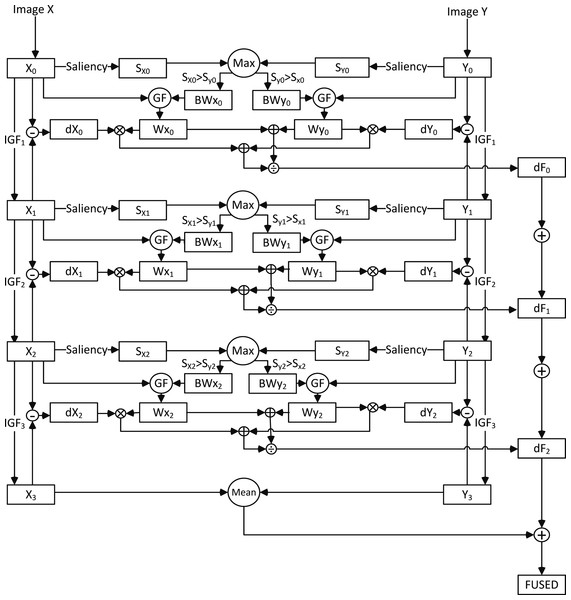Figure 1: Flow chart of the proposed image fusion scheme. The processing scheme is illustrated for two source images X and Y and 4 resolution levels (0–3). X0 and Y0 are the original input images, while Xi and Yi represent successively lower resolution versions obtained by iterative guided filtering. ‘Saliency’ represents the frequency-tuned saliency transformation, ‘Max’ and ‘Mean’ respectively denote the pointwise maximum and mean operators, ‘(I)GF’ means (Iterative) Guided Filtering, ‘dX,’ ‘dY’ and ‘dF’ are respectively the original and fused detail layers, ‘BW’ the binary weight maps, and ‘W’ the smooth weight maps.   Download full-size image DOI: 10.7717/peerjcs.80/fig-1

## Proposed Method

A flow chart of the proposed multi-scale decomposition fusion scheme is shown in Fig. 1. The algorithm consists of the following steps:

• 1.

Iterative guided filtering is applied to decompose the source images into approximate layers (representing large scale variations) and residual layers (containing small scale variations).

• 2.

Frequency-tuned filtering (Achanta et al., 2009) is used to generate saliency maps for the source images.

• 3.

Binary weighting maps are computed as the pixelwise maximum of the individual source saliency maps.

• 4.

Guided filtering is applied to each binary weighting map with its corresponding source as the guidance image to reduce noise and to restore spatial consistency.

• 5.

The fused image is computed as a weighted recombination of the individual source residual layers.

In a hierarchical framework steps 1–4 are performed at multiple spatial scales. In this paper we used a 4 level decomposition obtained by filtering at three different spatial scales (see Fig. 1).

Figure 2 shows the intensified visual (II) and thermal infrared (IR) or near infrared (NIR) images together with the results of the proposed image fusion scheme, for the 12 different scenes that were used in the present study. We will now discuss the proposed fusion scheme in more detail.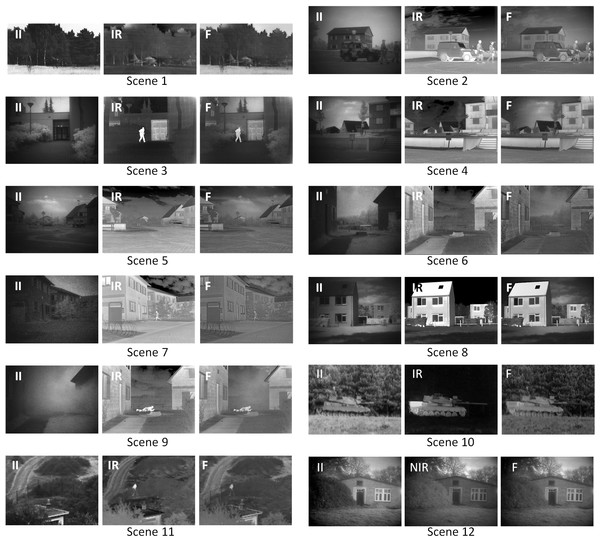Figure 2: Original input and fused images for all 12 scenes. The intensified visual (II), thermal infrared (IR) or near infrared (NIR: scene 12) source images together with the result of the proposed fusion scheme (F) for each of the 12 scenes used in this study.   Download full-size image DOI: 10.7717/peerjcs.80/fig-2

Consider two co-registered source images X0(x, y) and Y0(x, y). The proposed scheme then applies iterative guided filtering (IGF) to the input images Xi and Yi to obtain progressively coarser image representations Xi+1 and Yi+1 (i > 0): $\text{IGF}\left({X}_{i},{r}_{i},{\epsilon }_{i}\right)={X}_{i+1};\phantom{\rule{10.00002pt}{0ex}}i\in \left\{0,1,2\right\}$ where the parameters εi and ri represent respectively the range and the spatial variances of the guided filter at iteration step i. In this study the number of iteration steps is set to 4. By letting each finer scale image serve as the approximate layer for the preceding coarser scale image the successive size-selective residual layers dXi are simply obtained by subtraction as follows: $d{X}_{i}={X}_{i}-{X}_{i+1};\phantom{\rule{10.00002pt}{0ex}}i\in \left\{0,1,2\right\}.$ Figure 3 shows the approximate and residual layers that are obtained this way for the tank scene (nr 10 in Fig. 2). The edge-preserving properties of the iterative guided filter guarantee a graceful decomposition of the source images into details at different spatial scales. The filter size and regularization parameters used in this study are respectively set to ri = {5, 10, 30} and εi = {0.0001, 0.01, 0.1} for i = {0, 1, 2}.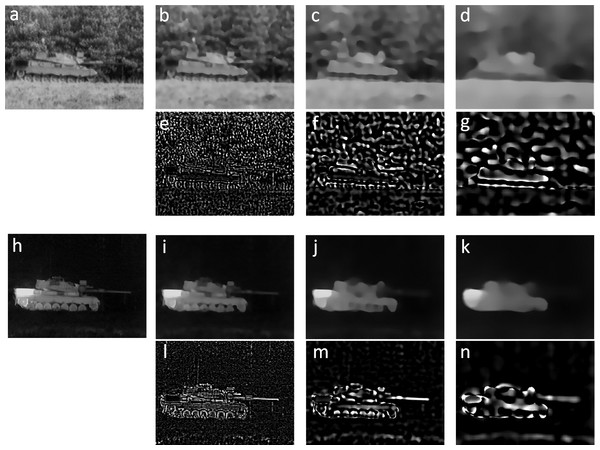Figure 3: Base and detail layers for the tank scene. Original intensified visual (A) and thermal infrared (H) images for scene nr. 10, with their respective base B–D and I–K and detail E–G and L–N layers at successively lower levels of resolution.   Download full-size image DOI: 10.7717/peerjcs.80/fig-3

Visual saliency refers to the physical, bottom-up distinctness of image details (Fecteau & Munoz, 2006). It is a relative property that depends on the degree to which a detail is visually distinct from its background (Wertheim, 2010). Since saliency quantifies the relative visual importance of image details saliency maps are frequently used in the weighted recombination phase of multi-scale image fusion schemes (Bavirisetti & Dhuli, 2016b; Cui et al., 2015; Gan et al., 2015). Frequency tuned filtering computes bottom-up saliency as local multi-scale luminance contrast (Achanta et al., 2009). The saliency map S for an image I is computed as $S\left(x,y\right)=∥{\mathbit{I}}_{\mu }-{\mathbit{I}}_{f}\left(x,y\right)∥$ where Iμ is the arithmetic mean image feature vector, If represents a Gaussian blurred version of the original image, using a 5 × 5 separable binomial kernel, ∥ ∥ is the L2 norm (Euclidian distance), and x, y are the pixel coordinates.

A recent and extensive evaluation study comparing 13 state-of-the-art saliency models found that the output of this simple saliency model correlates more strongly with human visual perception than the output produced by any of the other available models (Toet, 2011).

In the proposed fusion scheme we first compute saliency maps SXi and SYi for the individual source layers Xi and Yi, i ∈ {0, 1, 2}. Binary weight maps BWXi and BWYi are then computed by taking the pixelwise maximum of corresponding saliency maps SXi and SYi: $\begin{array}{c}B{W}_{{X}_{i}}\left(x,y\right)=\left\{\begin{array}{cc}1\hfill & \mathrm{if}{S}_{{X}_{i}}\left(x,y\right)>{S}_{{Y}_{i}}\left(x,y\right)\hfill \\ 0\hfill & \text{otherwise}\hfill \end{array}\right\\hfill \\ B{W}_{{Y}_{i}}\left(x,y\right)=\left\{\begin{array}{cc}1\hfill & \mathrm{if}{S}_{{Y}_{i}}\left(x,y\right)>{S}_{{X}_{i}}\left(x,y\right)\hfill \\ 0\hfill & \text{otherwise}.\hfill \end{array}\right\\hfill \end{array}$ The resulting binary weight maps are noisy and typically not well aligned with object boundaries, which may give rise to artefacts in the final fused image. Spatial consistency is therefore restored through guided filtering (GF) of these binary weight maps with the corresponding source layers as guidance images: $\begin{array}{c}{W}_{{X}_{i}}=\text{GF}\left(B{W}_{{X}_{i}},{X}_{i}\right)\hfill \\ {W}_{{Y}_{i}}=\mathrm{GF}\left(B{W}_{{Y}_{i}},{Y}_{i}\right).\hfill \end{array}$ As noted before guided filtering combines noise reduction with edge preservation, while the output is locally approximately a scaled version of the guidance image. In the present scheme these properties are used to transform the binary weight maps into smooth continuous weight maps through guided filtering with the corresponding source images as guidance images. Figure 4 illustrates the process of computing smoothed weight maps by guided filtering of the binary weight maps resulting from the pointwise maximum of the corresponding source layer saliency maps for the tank scene.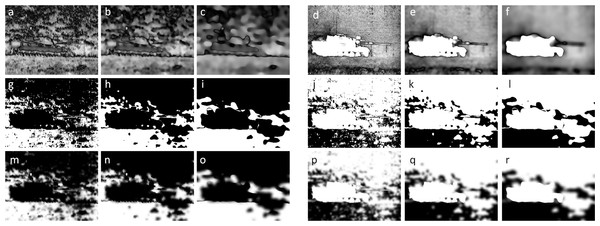Figure 4: Computing smoothed weight maps by guided filtering of binary weight maps. Saliency maps at levels 0, 1 and 2 for respectively the intensified visual (A–C) and thermal infrared (D–F) images from Fig. 3. Complementary binary weight maps for both image modalities (G–I and J–L) are obtained with a pointwise maximum operator at corresponding levels. Smooth continuous weight maps (M–O and P–R) are produced by guided filtering of the binary weight maps with their corresponding base layers as guidance images.   Download full-size image DOI: 10.7717/peerjcs.80/fig-4

Fused residual layers are then computed as the normalized weighted mean of the corresponding source residual layers: $d{F}_{i}=\frac{{W}_{{X}_{i}}\cdot d{X}_{i}+{W}_{{Y}_{i}}\cdot d{Y}_{i}}{{W}_{{X}_{i}}+{W}_{{Y}_{i}}}.$ The fused image F is finally obtained by adding the fused residual layers to the average value of the coarsest source layers: $F=\frac{{X}_{3}+{Y}_{3}}{2}+\sum _{i=0}^{2}d{F}_{i}.$ By using guided filtering both in the decomposition stage and in the recombination stage, this proposed fusion scheme optimally benefits from both the multi-scale edge-preserving characteristics (in the iterative framework) and the structure restoring capabilities (through guidance by the original source images) of the guided filter. The method is easy to implement and computationally efficient.

## Methods and Material

This section presents the test imagery and computational metrics used to assess the performance of the proposed images fusion scheme in comparison to existing multi-scale fusion schemes.

### Test imagery

Figure 2 shows the intensified visual (II), thermal infrared (IR) or near infrared (NIR: scene 12) source images together with the result of the proposed fusion scheme (F) for each of the 12 scenes used in this study. The 12 scenes are part of the TNO Image Fusion Dataset (Toet, 2014) with the following identifiers: airplane_in_trees, Barbed_wire_2, Jeep, Kaptein_1123, Marne_07, Marne_11, Marne_15, Reek, tank, Nato_camp_sequence, soldier_behind_smoke, Vlasakkers.

### Multi-scale fusion schemes used for comparison

In this study we compare the performance of our image fusion scheme with seven other popular image fusion methods based on multi-scale decomposition including the Laplacian pyramid (Burt & Adelson, 1983), the Ratio of Low-Pass pyramid (Toet, 1989b), the contrast pyramid (Toet, Van Ruyven & Valeton, 1989), the filter-subtract-decimate Laplacian pyramid (Burt, 1988; Burt & Kolczynski, 1993), the gradient pyramid (Burt, 1992; Burt & Kolczynski, 1993), the morphological pyramid (Toet, 1989a), the discrete wavelet transform (Lemeshewsky, 1999; Li, Manjunath & Mitra, 1995; Li, Kwok & Wang, 2002; Scheunders & De Backer, 2001), and a shift invariant extension of the discrete wavelet transform (Lemeshewsky, 1999; Rockinger, 1997; Rockinger, 1999; Rockinger & Fechner, 1998). We used Rockinger’s freely available Matlab image fusion toolbox (www.metapix.de/toolbox.htm) to compute these fusion schemes. To allow a straightforward comparison, the number of scale levels is set to 4 in all methods, and simple averaging is used to compute the approximation of the fused image representation at the coarsest spatial scale. Figures 59 show the results of the proposed method together with the results of other seven fusion schemes for some of the scenes used in this study (scenes 2–5 and 10).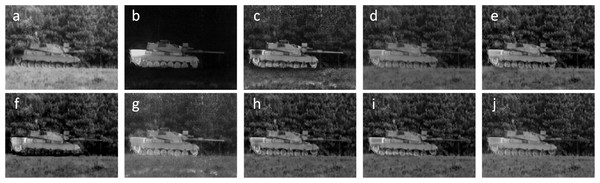Figure 5: Comparison with existing multiresolution fusion schemes. Original intensified visual (A) and thermal infrared (B) images for scene nr 10, and the fused results obtained with respectively a Contrast Pyramid (C), Gradient Pyramid (D), Laplace Pyramid (E), Morphological Pyramid (F), Ratio Pyramid (G), DWT (H), SIDWT (I), and the proposed method (J), for scene nr. 10.   Download full-size image DOI: 10.7717/peerjcs.80/fig-5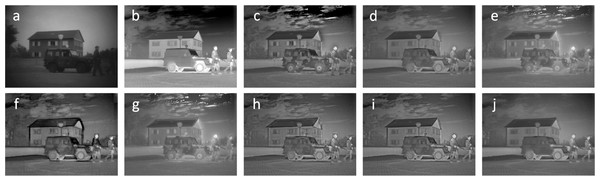Figure 6: As Fig. 5, for scene nr. 2.   Download full-size image DOI: 10.7717/peerjcs.80/fig-6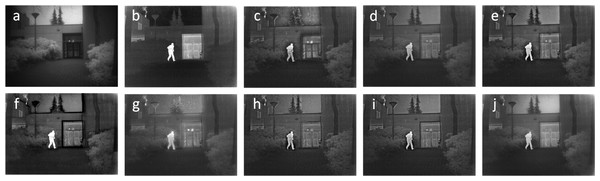Figure 7: As Fig. 5, for scene nr. 3.   Download full-size image DOI: 10.7717/peerjcs.80/fig-7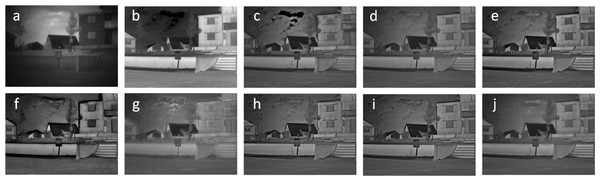Figure 8: As Fig. 5, for scene nr. 4.   Download full-size image DOI: 10.7717/peerjcs.80/fig-8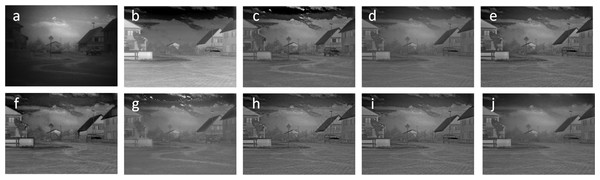Figure 9: As Fig. 5, for scene nr. 5.   Download full-size image DOI: 10.7717/peerjcs.80/fig-9

### Objective evaluation metrics

Image fusion results can be evaluated using either subjective or objective measures. Subjective methods are based on psycho-visual testing and are typically expensive in terms of time, effort, and equipment required. Also, in most cases, there is only little difference among fusion results. This makes it difficult to subjectively perform the evaluation of fusion results. Therefore, many objective evaluation methods have been developed (for an overview see e.g., Li, Li & Gong, 2010; Liu et al., 2012). However, so far, there is no universally accepted metric to objectively evaluate the image fusion results. In this paper, we use four frequently applied computational metrics to objectively evaluate and compare the performance of different image fusion methods. The metrics we use are Entropy, the Mean Structural Similarity Index (MSSIM), Normalized Mutual Information (NMI), and Normalized Feature Mutual Information (NFMI). These metrics will be briefly discussed in the following sections.

#### Entropy

Entropy (E) is a measure of the information content in a fused image F. Entropy is defined as ${E}_{F}=-\sum _{i=0}^{L-1}{P}_{F}\left(i\right)log{P}_{F}\left(i\right)$ where PF(i) indicates the probability that a pixel in the fused image F has a gray value i, and the gray values range from 0 to L. The larger the entropy is, the more informative the fused image is. A fused image is more informative than either of its source images when its entropy is higher than the entropy of its source images.

#### Mean Structural Similarity Index

The Structural Similarity (SSIM: Wang et al., 2004) index is a stabilized version of the Universal Image Quality Index (UIQ: Wang & Bovik, 2002) which can be used to quantify the structural similarity between a source image A and a fused image F: ${\mathrm{SSIM}}_{x,y}=\frac{2{\mu }_{x}{\mu }_{y}+{C}_{1}}{{\mu }_{x}^{2}+{\mu }_{y}^{2}+{C}_{1}}\cdot \frac{2{\sigma }_{x}{\sigma }_{y}+{C}_{2}}{{\sigma }_{x}^{2}+{\sigma }_{y}^{2}+{C}_{2}}\cdot \frac{{\sigma }_{xy}+{C}_{3}}{{\sigma }_{x}{\sigma }_{y}+{C}_{3}}$ where x and y represent local windows of size M × N in respectively A and F, and ${\mu }_{x}=\frac{1}{M×N}\sum _{i=1}^{M}\sum _{j=1}^{N}x\left(i,j\right),\phantom{\rule{10.00002pt}{0ex}}{\mu }_{y}=\frac{1}{M×N}\sum _{i=1}^{M}\sum _{j=1}^{N}y\left(i,j\right)$ ${\sigma }_{x}^{2}=\frac{1}{M×N}\sum _{i=1}^{M}\sum _{j=1}^{N}{\left(x\left(i,j\right)-{\mu }_{x}\right)}^{2},\phantom{\rule{10.00002pt}{0ex}}{\sigma }_{y}^{2}=\frac{1}{M×N}\sum _{i=1}^{M}\sum _{j=1}^{N}{\left(y\left(i,j\right)-{\mu }_{y}\right)}^{2}$ ${\sigma }_{xy}^{2}=\frac{1}{M×N}\sum _{i=1}^{M}\sum _{j=1}^{N}\left(x\left(i,j\right)-{\mu }_{x}\right)\left(y\left(i,j\right)-{\mu }_{y}\right).$ By default, the stabilizing constants are set to C1 = (0.01⋅L)2, C2 = (0.03⋅L)2 and C3 = C2∕2, where L is the maximal gray value. The value of SSIM is bounded and ranges between −1 and 1 (it is 1 only when both images are identical). The SSIM is typically computed over a sliding window to compare local patterns of pixel intensities that have been normalized for luminance and contrast. The Mean Structural Similarity (MSSIM) index quantifies the overall similarity between a source image A and a fused image F: ${\mathrm{MSSIM}}_{A,F}=\frac{1}{{N}_{w}}\sum _{i=1}^{{N}_{w}}{\mathrm{SSIM}}_{{x}_{i},{y}_{i}}$ where Nw represents the number of local windows of the image. An overall image fusion quality index can then be defined as the mean MSSIM values between each of the source images and the fused result: ${\mathrm{MSSIM}}_{F}^{A,B}=\frac{{\mathrm{MSSIM}}_{A,F}+{\mathrm{MSSIM}}_{B,F}}{2}$ ${\mathrm{MSSIM}}_{F}^{A,B}$ranges between −1 and 1 (it is 1 only when both images are identical).

#### Normalized Mutual Information

Mutual Information (MI) measures the amount of information that two images have in common. It can be used to quantify the amount of information from a source image that is transferred to a fused image (Qu, Zhang & Yan, 2002). The mutual information MIAF between a source image A and a fused image F is defined as: ${\mathrm{MI}}_{A,F}=\sum _{i,j}{P}_{A,F}\left(i,j\right)log\frac{{P}_{A,F}\left(i,j\right)}{{P}_{A}\left(i\right){P}_{F}\left(j\right)}$ where PA(i) and PF(j) are the probability density functions in the individual images, and PAF(i, j) is the joint probability density function.

The traditional mutual information metric is unstable and may bias the measure towards the source image with the highest entropy. This problem can be resolved by computing the normalized mutual information (NMI) as follows (Hossny, Nahavandi & Creighton, 2008): ${\mathrm{NMI}}_{F}^{A,B}=\frac{{\mathrm{MI}}_{A,F}}{{H}_{A}+{H}_{F}}+\frac{{\mathrm{MI}}_{B,F}}{{H}_{B}+{H}_{F}}$ where HA, HB and HF are the marginal entropy of A, B and F, and MIA,F and MIB,F represent the mutual information between respectively the source image A and the fused image F and between the source image B and the fused image F. A higher value of NMI indicates that more information from the source images is transferred to the fused image. The NMI metric varies between 0 and 1.

#### Normalized Feature Mutual Information

The Feature Mutual Information (FMI) metric calculates the amount of image features that two images have in common (Haghighat & Razian, 2014; Haghighat, Aghagolzadeh & Seyedarabi, 2011). This method outperforms other metrics (e.g., E, NMI) in consistency with the subjective quality measures. Previously proposed MI-based image fusion quality metrics use the image histograms to compute the amount of information a source and fused image have in common (Cvejic, Canagarajah & Bull, 2006; Qu, Zhang & Yan, 2002). However, image histograms contain no information about local image structure (spatial features or local image quality) and only provide statistical measures of the number of pixels in a specific gray-level. However, since meaningful image information is contained in visual features, image fusion quality measures should measure the extent to which these visual features are transferred into the fused image from each of the source images. The Feature Mutual Information (FMI) metric calculates the mutual information between image feature maps (Haghighat & Razian, 2014; Haghighat, Aghagolzadeh & Seyedarabi, 2011). A typical image feature map is for instance the gradient map, which contains information about the pixel neighborhoods, edge strength and directions, texture and contrast. Given two source images as A and B and their fused image as F, the FMI metric first extracts feature maps of the source and fused images using a feature extraction method (e.g., gradient). After feature extraction, the feature images A′, B′ and F′ are normalized to create their marginal probability density functions PA, PB and PF. The joint probability density functions PA′,F and PB′,F are then estimated from the marginal distributions using Nelsen’s method (Nelsen, 1987). The algorithm is described in more detail elsewhere (Haghighat, Aghagolzadeh & Seyedarabi, 2011). The FMI metric between a source image A and a fused image F is then given by ${\mathrm{FMI}}_{A,F}={\mathrm{MI}}_{{A}^{\prime },{F}^{\prime }}=\sum _{i,j}{P}_{{A}^{\prime },{F}^{\prime }}\left(i,j\right)log\frac{{P}_{{A}^{\prime },{F}^{\prime }}\left(i,j\right)}{{P}_{{A}^{\prime }}\left(i\right){P}_{{F}^{\prime }}\left(j\right)}$ and the normalized feature mutual information (FMI) can be computed as follows ${\mathrm{FMI}}_{F}^{A,B}=\frac{{\mathrm{MI}}_{{A}^{\prime },{F}^{\prime }}}{{H}_{{A}^{\prime }}+{H}_{{F}^{\prime }}}+\frac{{\mathrm{MI}}_{{B}^{\prime },{F}^{\prime }}}{{H}_{{B}^{\prime }}+{H}_{{F}^{\prime }}}.$ In practice the FMI is computed locally over small corresponding windows between the source and the fused images and averaged over all windows covering the image plane (Haghighat & Razian, 2014).

## Results

### Fusion evaluation

Here we assess the performance of the proposedimage fusion scheme on the intensified visual and thermal infrared images for each of the 12 selected scenes, using Entropy, the Mean Structural Similarity Index (MSSIM), Normalized Mutual Information (NMI), and Normalized Feature Mutual Information (NFMI) as the objective performance measures. We also compare the results of the proposed method with those of seven other popular multi-scale fusion schemes.

Table 1 lists the entropy of the fused result for the proposed method (IGF) and all seven multi-scale comparison methods (Contrast Pyramid, DWT, Gradient Pyramid, Laplace Pyramid, Morphological Pyramid, Ratio Pyramid, SIDWT). It appears that IGF produces a fused image with the highest entropy for 9 of the 12 test scenes. Note that a larger entropy implies more edge information, but it does not mean that the additional edges are indeed meaningful (they may result from over enhancement or noise). Therefore, we also need to consider structural information metrics.

Table 2 shows that IGF outperforms all other multi-scale methods tested here in terms of MSSIM. This means that the mean overall structural similarity between both source images the fused image F is largest for the proposed method.

Table 3 shows that IGF also outperforms all other multi-scale methods tested here in terms of NMI. This indicates that the proposed IGF fusion scheme transfers more information from the source images to the fused image than any of the other methods.

Table 4 shows that IGF also outperforms 10 of the 12 other multi-scale methods tested here in terms of NFMI. IGF is only outperformed by SIDWT for scene 1 and by the Contrast Pyramid for scene 7. This implies that fused images produced by the proposed IGF scheme typically have a larger amount of image features in common with their source images than the results of most other fusion schemes.

Summarizing, the proposed IGF fusion scheme appears to outperform the other multi-scale fusion methods investigated here in most of the conditions tested.

### Runtime

In this study we used a Matlab implementation of the GF and IGF written by Zhang et al. (2014) that is freely available from the authors (at http://www.cs.cuhk.edu.hk/~leojia/projects/rollguidance). We made no effort to optimize the code of the algorithms. We conducted a runtime test on a Dell Latitude laptop with an Intel i5 2 GHz CPU and 8 GB memory. The algorithms were implemented in Matlab 2016a. Only a single thread was used without involving any SIMD instructions. For this test we used the set of 12 test images described in ‘Test imagery.’ As noted before, the filter size and regularization parameters used in this study are respectively set to ri = {5, 10, 30} and εi = {0.0001, 0.01, 0.1} for spatial scale levels i = {0, 1, 2}. The mean runtime of the proposed fusion method was 0.61 ± 0.05 s.

## Discussion and Conclusions

We propose a multi-scale image fusion scheme based on guided filtering. Iterative guided filtering is used to decompose the source images into approximation and residual layers. Initial binary weighting maps are computed as the pixelwise maximum of the individual source saliency maps, obtained from frequency tuned filtering. Spatially consistent and smooth weighting maps are then obtained through guided filtering of the binary weighting maps with their corresponding source layers as guidance images. Saliency weighted recombination of the individual source residual layers and the mean of the coarsest scale source layers finally yields the fused image. The proposed multi-scale image fusion scheme achieves spatial consistency by using guided filtering both at the decomposition and at the recombination stage of the multi-scale fusion process. Application to multiband visual (intensified) and thermal infrared imagery demonstrates that the proposed method obtains state-of-the-art performance for the fusion of multispectral nightvision images. The method has a simple implementation and is computationally efficient.

## Additional Information and Declarations

### Competing Interests

The author declares there are no competing interests.

### Author Contributions

Alexander Toet conceived and designed the experiments, performed the experiments, analyzed the data, contributed reagents/materials/analysis tools, wrote the paper, prepared figures and/or tables, performed the computation work, reviewed drafts of the paper.

### Data Availability

The following information was supplied regarding data availability:

Figshare: TNO Image Fusion Dataset

### Funding

The effort was sponsored by the Air Force Office of Scientific Research, Air Force Material Command, USAF, under grant number FA9550-15-1-0433. The funders had no role in study design, data collection and analysis, decision to publish, or preparation of the manuscript.